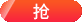C++函数模型template声明

template<typename 数据类型参数标识符>
< 返回类型><函数名>(参数表)

{
函数体
}

#include<iostream>
using namespace std;
template<typename T>
void Swap(T &a,T &b)
{
T c;
c=a;
a=b;
b=c;
}
int main()
{
int a=5;
int b=3;
Swap(a,b);
cout<<"a:"<<a<<" "<<"b:"<<b<<endl;

double c=1.2;
double d=3.6;
Swap(c,d);
cout<<"c:"<<c<<" "<<"d:"<<d<<endl;

system("pause");
}

a:3 b:5
c:3.6 d:1.2

#include<iostream>
using namespace std;
template<typename T1,typename T2>
T2 Add(T1 a,T2 b)
{
T2 c;
c = a+b;
return c;
}
int main()
{
int a=5;
double b=1.2;

double c=1.2;
int d = 5;

system("pause");
}

6.2
6

相关阅读